Also available in italiano

# Measuring the average speed of a comet

Created: 2022-07-08
Author(s): Giulia Iafrate, INAF; Giulia Pantiri, INAF; Riccardo Bevilacqua, INAF; Stefano Sandrelli, INAF

Using telescope images of comet C/2019 Y4 we calculate its average speed and understand what a physical quantity is.Goals
• Observe, describe, and qualitatively analyse the movement of a body
• Observe, describe, and quantitatively analyse the movement of a body
• Be aware of the representation of a phenomenon
• Be aware of the meaning of “measure” and “error” associated to measure
• Be aware of the meaning of the “estimate” of a quantity
• Be aware of the potential of technologies to represent the movement of a body
• Encourage critical-creative thoughtLearning Objectives

Students, in the course of the activity, will have the following specific learning goals:

• Use of a software for viewing the representation of the movement of a body;
• Representation of the phenomenon through tables and charts;
• Use of scale factors;
• Facing the problem of measuring an observed quantity;
• Critical discussion of the hypothesis.Background

### What is a comet?

Comets are celestial objects composed of rocks and ice, which orbit around the Sun, travelling along regular paths, which bring them even further away from our star. As they approach the Sun, the layers of ice which cover them are heated and partially sublimate, producing a cloud of gas, from which originates the well-known cometary tail, which, in rare circumstances, can be visible to the naked eye from the Earth.

The Comet C/2019 Y4
The programme ATLAS (Asteroid Terrestrial-impact Last Alert System), active since December 2015, is in charge of identifying the small bodies of the Solar System, which collide with the Earth. ATLAS currently makes use of 8 50-cm-telescopes.On 28 December 2019, the programme observed a comet, which was about 450 millions km away from the Sun. The comet was called C/2019 Y4. Considered as highly visible, around 2 April, the comet suffered a process of fragmentation which caused a decrease of brightness, such that it could not be observed with a naked eye.

At the time of the observations, the comet was 155 434 400 km, about 155,5 millions km from the Sun.

The images of this activity were collected through the 14-inch Schmidt-Cassegrain telescope of the school project Le Stelle Vanno A Scuola (SVAS – Stars go to School) of INAF-Astronomical Observatory of Trieste.

### Speed values in daily life

In this table, we report a few values, typical of various kinds of speed we find in daily life. You can use this table to compare these values with the average speed of the comet.

che cosa m/s km/h
Corsa tranquilla 2 7,2
Record del mondo sui 100 metri 10,44 37,58
Treno freccia rossa 83,3 300
Aereo 3 ×10 2 1080
Suono nel vetro 5 ×10 3 18000
Moto orbitale della Terra 3 ×10 4 108000Materials

Activity with a personal computer

• personal computer (1 every 2-3 students, or 1 only, according to the chosen modality)
• Free Software - SalsaJ – installed on the computer

Activity with printed material If you prefer to carry out the activity with paper materials, you can print the pictures. In this case, you will need:

• a printer
• spreadsheet or graph paper and a calculator
• paper sheets, pen or pencil, and a rulerFull Description

We provide 12 images of the same celestial field (see attached files: ImmaginiATLAS): a comet is visible in the foreground, on the right hand-side of the image, with a background of stars (in the centre you can also see a galaxy). The images are centred and aligned with each other. Each image shows the start time. The duration of the pose is 60 seconds.The images are numbered 0001, 0005, 0010, 0015 and so on, in chronological order.

Overlapping the complete set of images collected, we have provided a video (see attached files: CometaAtlas Video), in which the movement of the comet has a twofold factor of representation:- an arbitrary timescale, decided during the assembly;- an intrinsic space-scale: to a certain length in mm, covered by the comet in the background of fixed stars, corresponds a certain distance in km.

The purpose of this activity is the measurement of the average speed of the comet C/2019 Y4 in the course of the observations of 2 April, made through SVAS. The average speed will be measured in relation to a reference system attached to the observation point.

By definition, the average speed of a body is the relationship between its movement and the interval of time Δt in which it occurred:

v media = d / Δt

It will be therefore necessary to derive both terms from the images:

d = length of the comet’s movement;

Δt = duration of the time interval of the movement

Finally, we will discuss limits and approximations of the measurement made.

In the course of the activity, we will also detect the trajectory of the comet in the interval of observation (optional).

### Preparation

We will measure the coordinates of the comet in the images provided. To this aim, we can use the free software SalsaJ, which you can download at this link .

Otherwise, you can print the images, making sure that they are all printed with the same scale.

Step 1: watching the videoIn this short video, the sequence of positions of C/2019 Y4 during the observations is shown. Note its quick movement, with respect to the stellar field, i.e. the background of stars appearing in the images.

Step 2: duration of the movementIn order to derive the total time-duration of the observations Δt, you should take into account the following data: - the first observation started at 22:08:12 UT- the last observation ended at 23:32:42 UT.

It will be useful to express the duration of observations in seconds.

Optional. You can also derive the factor of time scale of the video, i.e. how many real seconds correspond to a second of video. In order to do that, you should simply watch the video and measure its duration: Factor of time scale = duration of observations/duration of the video

Answers Duration of the video = 5,5 secondsTotal duration of the observations: 1 hour, 24 minutes and 30 seconds, or 5070 sFactor of time scale = 922, i.e. each second of the video corresponds to about 922 real seconds (15 minutes 22 seconds)

Step 3: the measure of the comet’s position in an astronomical image.In the following, we describe a possible procedure to measure the comet’s position in one of the images provided. The procedure is valid in case you use the software SalsaJ, but the method can easily be adapted to activities with printed material, or with any other software capable of automatically counting pixels in the image.

By launching the application SalsaJ, this window will appear.Upload the astronomical image (or images) provided. You can do this in two ways: a) by using the command Open File Image of SalsaJ (first icon on the top left) and selecting the images provided; b) opening the folder and dragging the images on the program itself. Scroll the cursor on an image and note that, as it moves, three indicators in the mask of SalsaJ move down on the left:

x = increases from left to right

y = increases top down

value = can assume a value from 0 to 255 and increases moving from black areas to light areas. This is a parameter which indicates the brightness of a star.

The coordinates (x; y), expressed in pixels, form a system of coordinates on the plane, whose origin (0;0) is fixed in the top-left corner of the images. This is a choice of the programmers of SalsaJ. In doing so, you will see that you obtain a system of cartesian axes, like the one in figure 1.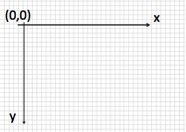Starting from the image number 0001, move the cursor on the comet. We will associate the position of the comet to the coordinates of pixels corresponding to the top value (related to the surrounding values) of the brightness parameter.

Note that with this method, position determination is not unique: for each image, there are adjacent pixels, both along the x axis and along the y axis, in which the value of brightness is equally high. According to the level of difficulty you want to keep, you can decide to ignore this effect, or you can associate to each measure an uncertainty of a few pixels.

Answer. For image 001, a possible measure is (we will use the notation +/- with the mathematical meaning of “plus or minus”):

x = 887 +/- 4 y = 409 +/- 3

Step 4: the trajectory of the comet. Note: if you want to use this activity only to define the average speed, you can proceed directly to step 5.

Repeat the procedure in order to detect the position of the comet for each image, associating with C/2019 Y4 a position in each image. You can also add two further lines for uncertainties Δx and Δy. You will thus obtain the table (x,y) for the comet’s motion.

Let us place the data on a chart through a spreadsheet, or graph paper, thus obtaining an image like the one in figure 2, in which we highlighted the error bars: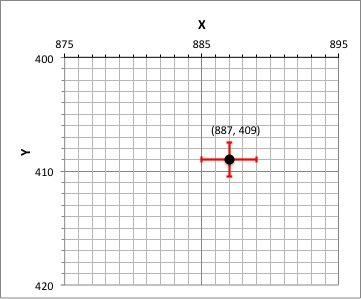In case you use a spreadsheet, the table should be like the one above, with aligned data, and the type of chart should be “a scatter plot”.

In both cases, you should pay attention to two details: If you want to represent a trajectory, you should use the same scale on both axes, so as not to introduce deformation factors. Once we have the numerical values to enter, we should shift the origin of the axes.

With reference to the above-mentioned values, we obtain a simple linear chart. Check the alignment of data: this activity can be particularly interesting if you have decided to associate an error to the measurements of position.

Answer: for the table, the following is a possible result

Immagini 1 2 3 4 5 6 7 8 9 10 11 12
x 887 875 860 846 830 814 799 783 767 755 739 724
y 409 407 407 403 401 399 398 396 396 391 389 387
Δx 4 4 4 4 4 4 4 4 4 4 4 4
Δy 3 3 3 3 3 3 3 3 3 3 3 3

Data can be represented as in the following figure, where we also reported a trend line.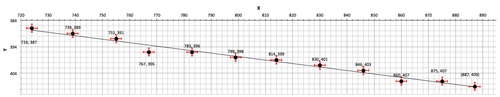Step 5: the space covered by the comet in the course of observationsAt the distance of the comet, each pixel corresponds to 620 km:

1 px = 620 km

This value is obtained by a method which is outside the aims of this activity, which assumes to know the distance of the comet with a good approximation.This hypothesis simplifies this activity, but may be a considerable source of error.

The scale factor is confirmed in image #1, where we reported a 20-pixel white line. It will serve in case you decide to realise the activity by printing the images: from this direction you can derive the scale between centimetres on paper and km – which obviously depend on the dimensions you have chosen for printing.

In order to measure the distance d covered by the comet in the interval Δt we have considered, it is necessary to calculate the movement between its initial and final position. We will then measure the coordinates of the comet in the image 001 (initial position) and in the image 055 (final position). Applying the theorem of Pythagoras, we will calculate the distance d through the coordinates of the points, thus obtaining a value expressed in pixels: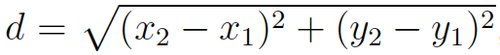In order to calculate the distance in km, you should multiply the value of the distance in pixels for the above scale factor.

Answer. With these data you obtain:

Initial position (x1, y1) = (887 +/- 4 ; 409 +/- 3) Final position (x2, y2) = (724 +/- 4 ; 387 +/- 3)

distance: d (px) = 165 +/- 9 pixel

The distance covered in pixels corresponds to a total of 102300 km, whereas the error is 5580 km. By taking into account significant figures, the result can be expressed as:

d (km) = 102000 +/- 6000 km

with a relative error of Δd/d = 0,06

Step 6: the definition and calculation of the average speed. The average speed of a body is, by definition, the relationship between the distance covered and the interval of time Δt employed:

vmedia = d / Δt

Both values are known. We suggest that you always use the SI of the unit of measurement, expressing the speed in metres per second (m/s), or, if you want a direct comparison with types of speed familiar in daily life, in km/h. We can overlook the error in the interval of time. Even assuming an absolute error of 10 seconds, the relative error is much smaller than the one in the measurement of the distance covered:

Δt/t = 10 s / 5070 s = 0,002

Solution. Using the data obtained above, we discover that the average speed of the comet C/2019 Y4, on 2 april, was about:

v media = 20 000 m/s = 72400 km/h

with a relative error of Δ vmedia / vmedia = 0,06 you obtain

v media = (72000 +/- 4000) km/h

### Discussion

Discussion 1: direction of the movement of the cometWe have carried out the activity on the basis of an implicit hypothesis, which we did not express, and yet we should discuss it in order to understand the meaning itself of the estimate. The images we have analysed show the projection of the movement of the comet with the background of the sky, which appears – by definition – perpendicular to our line of sight: also the photos we take every day, being two-dimensional, project the subject onto the background. Only if the trajectory of the comet were actually perpendicular to our line of sight, our chart would correspond to the real trajectory. It would be a particular and lucky case. In general, indeed, the direction of movement of the comet will not be perpendicular to the line of sight: in these cases, our measurement does not correspond to the real value of the comet speed, but rather to the value of one of its components. Our measure, in sum, is a lower speed limit value: the speed of the comet cannot be less than we have estimated.

Discuss this problem: what is the distance between the comet and the stars in the background?

Discussion 2: sources of error and their estimateIn order to simplify the activity, we have considered the following misure subject to a negligible error:the measure of the comet distance; the measure of time. Our assumption is doubtless correct in the second case, but the measure of the comet distance may be a source of non-negligible error, because it is reflected on the conversion pixel → km of the linear scale on images, and therefore on the distance which has effectively been covered.

On the other hand, we have quantified the errors associated with the measure of the comet’s position in the various images, and provided a plausible error on the measure of the distance covered. You can use the propagation of errors, even in simple form, in order to draw this value. In the following, we provide a short explanation of the procedure which should be used to calculate the final error. You can find an in-depth, detailed version of it in appendix 1. Since we have considered the error on Δt negligible, it will be enough to estimate the error on the distance covered d:By calculating separately the errors on each operation and, considering the propagation of errors in its simpler form, we obtain, with the data we provided: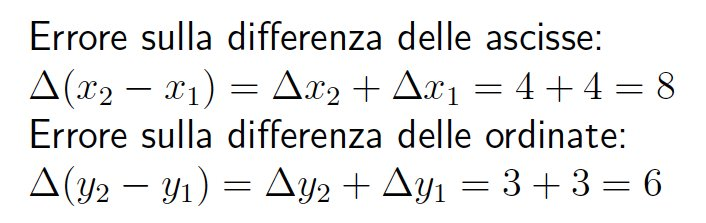The error in their squares is: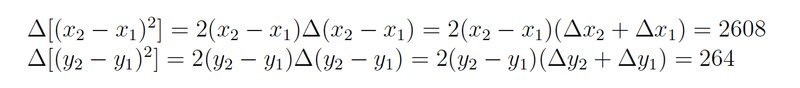Error in the sum of the squares: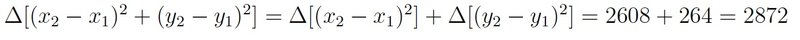Error in the square root: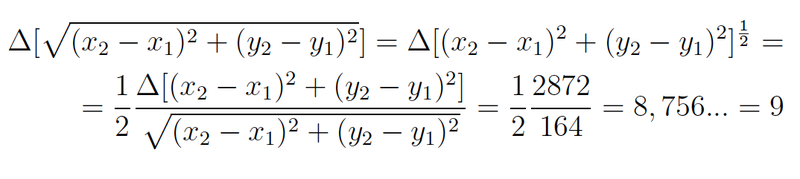Ultimately, the error in the measure of distance is Δd = 9 pixels.

Discussion 3: reference systemsPropose a discussion about the reference system adopted in this activity: against which reference system was measured the average speed of the comet? Which examples in the table concern the same reference system?

Discussion 4: comparison among the data of the groups (or students)Now we want to discuss the compatibility of measurements obtained by all the students of the class, by comparing them.You can fill a table/spreadsheet with the results obtained by each pupil (speed obtained and associated absolute error).Let us try and answer these questions together:

1. How can we represent data in a chart so as to verify their compatibility?
2. If they are not all compatible, how many of them are? Imagine a reason why some measures are not compatible with the others.
3. Calculate the average, with absolute and relative error, of compatible measurements.

Answers 1. On the chart, we insert in the axis of ordinates the speed (in km/h), and in the axis of abscisses a dummy variable, which ranks the measurements (for example each student is associated with a number in the school register). The measurements are all compatible among them, if you manage to draw at least one horizontal line which interpolates measures within error bars.

1. If they are not all compatible, the most probable reason is the mistake of some student in the procedure for calculating speed. In this case, finding out the measures which are not on the line (or in the horizontal band, constituted by the series of horizontal lines interpolating data) can help single out the students who did not obtain a correct result, and discuss with them the reasons for their result.
2. The answer to this question depends on how the issue of the theory of error has been addressed by the class. According to the correct theory, you need to calculate the weighted average, and, as an absolute error, the error in the weighted average. However, in order to simplify the concept, we often take the arithmetic mean, and as absolute error we take the semi-dispersal of data.Evaluation

The evaluation of learning can be qualitatively estimated in the course of the discussions 1-3 proposals. In this case, the object of evaluation is the procedure and the capacity to reflect on the method you use.

We suggest a quantitative evaluation through the comparison between the measure taken by groups and/or single students (see in particular discussion 4 )

Here are a few specific questions you could propose.

• The speed you calculated is the speed of the comet at every moment? Explain your answer
• If the average speed were calculated between two consecutive positions (images), how would the absolute error in its measure change? What about the relative error?
• (If the trajectory has been represented). With reference to the measured values, while taking into account the associated error, you will obtain a simple linear chart. The measurements allow you to unambiguously locate the line which represents the trajectory? Explain your answer.Curriculum
Livello scolastico Materia Argomento
Secondaria di secondo grado Fisica Definizione di velocità media
Secondaria di secondo grado Fisica Traiettoria
Secondaria di secondo grado Fisica Unità di misura (MKS ed astronomiche)
Secondaria di secondo grado Fisica Teoria degli errori (misure dirette e derivate)
Secondaria di secondo grado Matematica Grafici cartesianiConclusion

We calculate the average speed of a comet by using a set of photographic images, collected in an interval of time of about 90 minutes. We measure the position of the comet as compared to the background of fixed stars, and a known scale factor, in order to estimate the distance covered. We discuss the errors made, both in measurement and in the hypotheses. We apply the rules of the error propagation.Further Reading